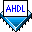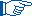## Defining Groups

 See AlsoA group is treated as a collection of nodes and acted upon as one unit. A group name can be specified with a single-range group name, dual-range group name, or sequential group name format.

In Boolean equations, a group can be set equal to a Boolean expression, another group, a single node, `VCC`, `GND`, 1, or 0. In each case, the value of the group is different. The Options Statement can be used to specify whether the lowest numbered bit of the group is the MSB, the LSB, or either.Once a group has been defined, `[]` is a shorthand way of specifying an entire range. For example, `a[4..1]` can also be denoted by `a[]`; similarly, `b[5..4][3..2]` can be represented by `b[][]`.

The group1.tdf file shows simple Boolean expressions that define multiple groups.

```OPTIONS BIT0 = MSB;
CONSTANT MAX_WIDTH = 1+2+3-3-1;  % MAX_WIDTH = 2 %
SUBDESIGN group1
(
a[1..2], use_exp_in[1+2-2..MAX_WIDTH]    : INPUT;
d[1..2], use_exp_out[1+2*2-4..MAX_WIDTH] : OUTPUT;
dual_range[5..4][3..2]                   : OUTPUT;
)
BEGIN
d[] = a[] + B"10";
use_exp_out[] = use_exp_in[];
dual_range[][] = VCC;
END;
```

In this example, the Options Statement is used to specify that the rightmost bit of each group is the MSB, and a `1` (decimal) is added to group `a[]`. If `00` is applied to input `a[]`, the result of this sample program is `d[] == 1` (decimal). The groups `use_exp_in[]` and `use_exp_out[]` show how constants and arithmetic expressions can be used to delimit group ranges.

The following examples illustrate group usage:

• When a group is set equal to another group of the same size, each member on the right is assigned to the member on the left that corresponds in position.  Example

• When a group is set equal to a single node, all bits of the group are connected to the node.  Example

• When a group is set equal to `VCC` or `GND`, all bits of the group are connected to that value.  Example

• When a group is set equal to `1` (decimal), only the LSB of the group is connected to the value `VCC`. All other bits in the group are connected to `GND`.  Example

• When a group is set equal to another group of a different size, the number of bits in the group on the left of the equation must be evenly divisible by the number of bits in the group on the right of the equation. The bits on the left of the equation are mapped to the right of the equation, in order.  Example